# Changing the contrast and brightness of an image!¶

## Goal¶

In this tutorial you will learn how to:

• Access pixel values
• Initialize a matrix with zeros
• Learn what saturate_cast does and why it is useful
• Get some cool info about pixel transformations

## Theory¶

Note

The explanation below belongs to the book Computer Vision: Algorithms and Applications by Richard Szeliski

### Image Processing¶

• A general image processing operator is a function that takes one or more input images and produces an output image.
• Image transforms can be seen as:
• Point operators (pixel transforms)
• Neighborhood (area-based) operators

#### Pixel Transforms¶

• In this kind of image processing transform, each output pixel’s value depends on only the corresponding input pixel value (plus, potentially, some globally collected information or parameters).
• Examples of such operators include brightness and contrast adjustments as well as color correction and transformations.
• Two commonly used point processes are multiplication and addition with a constant: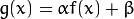• The parameters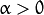and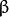are often called the gain and bias parameters; sometimes these parameters are said to control contrast and brightness respectively.

• You can think of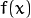as the source image pixels and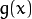as the output image pixels. Then, more conveniently we can write the expression as: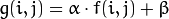whereandindicates that the pixel is located in the i-th row and j-th column.

## Code¶

• The following code performs the operation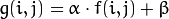:
#include <cv.h>
#include <highgui.h>
#include <iostream>

using namespace cv;

double alpha; /**< Simple contrast control */
int beta;  /**< Simple brightness control */

int main( int argc, char** argv )
{
/// Read image given by user
Mat image = imread( argv );
Mat new_image = Mat::zeros( image.size(), image.type() );

/// Initialize values
std::cout<<" Basic Linear Transforms "<<std::endl;
std::cout<<"-------------------------"<<std::endl;
std::cout<<"* Enter the alpha value [1.0-3.0]: ";std::cin>>alpha;
std::cout<<"* Enter the beta value [0-100]: "; std::cin>>beta;

/// Do the operation new_image(i,j) = alpha*image(i,j) + beta
for( int y = 0; y < image.rows; y++ )
{ for( int x = 0; x < image.cols; x++ )
{ for( int c = 0; c < 3; c++ )
{
new_image.at<Vec3b>(y,x)[c] =
saturate_cast<uchar>( alpha*( image.at<Vec3b>(y,x)[c] ) + beta );
}
}
}

/// Create Windows
namedWindow("Original Image", 1);
namedWindow("New Image", 1);

/// Show stuff
imshow("Original Image", image);
imshow("New Image", new_image);

/// Wait until user press some key
waitKey();
return 0;
}


## Explanation¶

1. We begin by creating parameters to save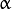andto be entered by the user:

double alpha;
int beta;

2. We load an image using imread and save it in a Mat object:

Mat image = imread( argv );

3. Now, since we will make some transformations to this image, we need a new Mat object to store it. Also, we want this to have the following features:

• Initial pixel values equal to zero
• Same size and type as the original image
Mat new_image = Mat::zeros( image.size(), image.type() );


We observe that Mat::zeros returns a Matlab-style zero initializer based on image.size() and image.type()

4. Now, to perform the operationwe will access to each pixel in image. Since we are operating with RGB images, we will have three values per pixel (R, G and B), so we will also access them separately. Here is the piece of code:

for( int y = 0; y < image.rows; y++ )
{ for( int x = 0; x < image.cols; x++ )
{ for( int c = 0; c < 3; c++ )
{ new_image.at<Vec3b>(y,x)[c] =
saturate_cast<uchar>( alpha*( image.at<Vec3b>(y,x)[c] ) + beta ); }
}
}


Notice the following:

• To access each pixel in the images we are using this syntax: image.at<Vec3b>(y,x)[c] where y is the row, x is the column and c is R, G or B (0, 1 or 2).
• Since the operation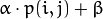can give values out of range or not integers (ifis float), we use saturate_cast to make sure the values are valid.
5. Finally, we create windows and show the images, the usual way.

namedWindow("Original Image", 1);
namedWindow("New Image", 1);

imshow("Original Image", image);
imshow("New Image", new_image);

waitKey(0);


Note

Instead of using the for loops to access each pixel, we could have simply used this command:

image.convertTo(new_image, -1, alpha, beta);


where convertTo would effectively perform new_image = a*image + beta. However, we wanted to show you how to access each pixel. In any case, both methods give the same result.

## Result¶

• Running our code and using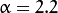and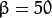\$ ./BasicLinearTransforms lena.jpg
Basic Linear Transforms
-------------------------
* Enter the alpha value [1.0-3.0]: 2.2
* Enter the beta value [0-100]: 50

• We get this: